# Syntax and new features in Java 8

#### View more Tutorials: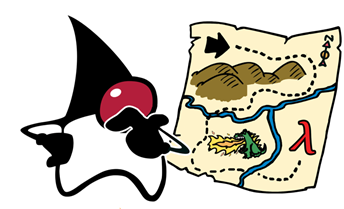## 1- Instroduction

From the invention to upgradation of syntax and features, Java has gone through some milestones:
• Java 1.0: Beginning of a programme language.
• Java 1.1, 1.2, 1.3, 1.4: there are not many changes in syntax and function.
• Java 1.5 (Or Java 5): there are big changes along with the addition of some new conceptions.
• Generic
• Autoboxing/Unboxing
• Upgrade the function of foreach.
• Type-safe enumerations.
• Varargs
• Static import
• Java 6,7 there is not big change in language.
• Java 8: there is a big change in language, along with the addition of some new concepts and functions:
• Default interface methods
• Lambda expressions
• Reference methods.
• Repeatable annotations
• Stream
In this post, I will introduce you to the features and syntax of Java 8.

## 2- Default methods for Interface

Java 8 enables us to add non-abstract method implementations to interfaces by utilizing the default keyword. This feature is also known as Extension Methods. Here is our first example:
Formula.java
```package org.o7planning.tutorial.j8.itf;

public interface Formula {

// Declare an abstract method.
double calculate(int a);

// Declare a method is not abstract.
// Use the keyword default.
// (Function calculate the square root of a number)
default double sqrt(int a) {
return Math.sqrt(a);
}

}
```
And class FormulaImpl implements interface Formula.
FormulaImpl.java
```package org.o7planning.tutorial.j8.itf;

// A class implements Formula interface.
public class FormulaImpl implements Formula {

// Just implements abstract method of Formula.
@Override
public double calculate(int a) {
return a * a - a;
}

}
```
FormulaTest.java
```package org.o7planning.tutorial.j8.itf;

public class FormulaTest {

public static void main(String[] args) {
Formula formula = new FormulaImpl();

// ==> 5
double value1 = formula.sqrt(25);
System.out.println("Value1 = " + value1);

// ==> 600
double value2 = formula.calculate(25);
System.out.println("Value2 = " + value2);

}

}
```

## 3- Functional Interface

Java8 considered the Interface has a single abstract method is the Functional Interface. You can use @FunctionalInterface annotation, to mark your interface is Functional Interface. It is not compulsory. However, the compiler of Java will notify you error if you add another abstract method into the interface marked by this annotation by mistake.
Here are some practical examples with @FunctionalInterface:
The example below is a valid FunctionalInterface because it has only one abstract method.
Foo.java
```package org.o7planning.tutorial.j8.funcitf;

@FunctionalInterface
public interface Foo {

void something();

default void defaultMethod() {
System.out.println("..");
}
}
```
Not valid: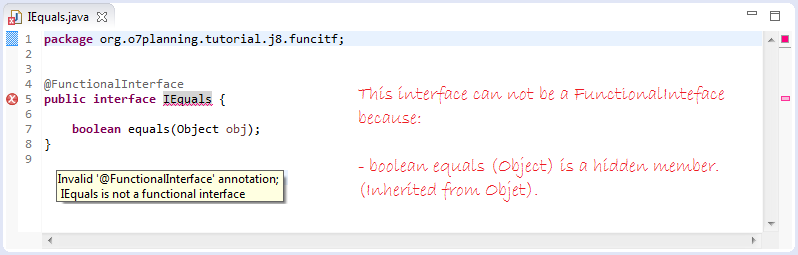Valid: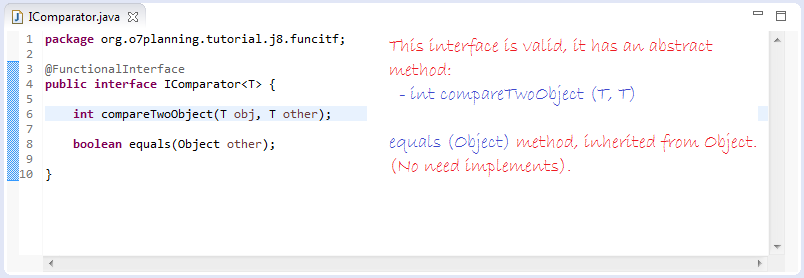Invalid: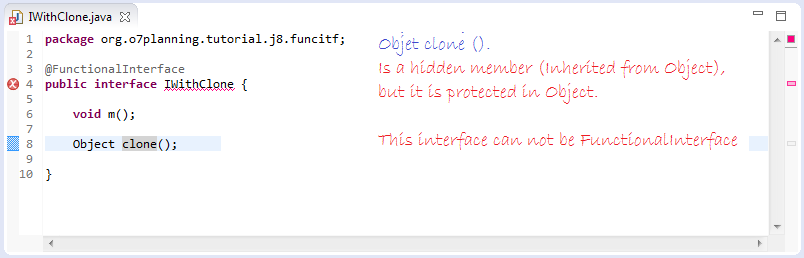Valid: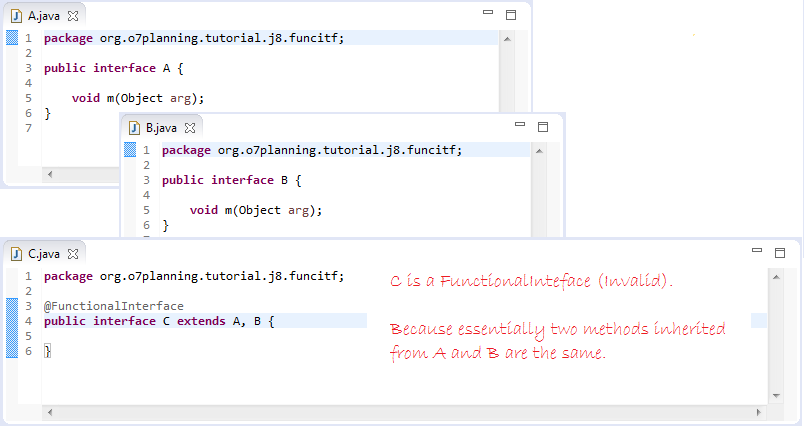## 4- Lambda Expression

First we will review how the Java version before 8 arrange a Collection.
See more:
SortBefore8Example.java
```package org.o7planning.tutorial.j8.lambda;

import java.util.Arrays;
import java.util.Collections;
import java.util.Comparator;
import java.util.List;

public class SortBefore8Example {

public static void main(String[] args) {

// A list of the fruits.
List<String> fruits = Arrays.asList("Grapefruit", "Apple", "Durian", "Cherry");

// Use utility method of Collections to sort a collection.
// Provide a Comparator.
Collections.sort(fruits, new Comparator<String>() {

@Override
public int compare(String o1, String o2) {
return o1.compareTo(o2);
}

});

for (String fruit : fruits) {
System.out.println(fruit);
}

}
}
```
Results of running the example above: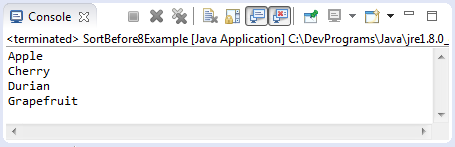Java 8 understands interfaces to have a unique abstract method which is  Functional Interface. Accordingly, when implementing the interface, you only need to write a method implements that unique abstract method.  Comparator is an interface having a unique abstract method, and it is a  Functional Interface. You can rewrite the above example in the form of   Lambda of  Java 8:
SortJava8Example.java
```package org.o7planning.tutorial.j8.lambda;

import java.util.Arrays;
import java.util.Collections;
import java.util.List;

public class SortJava8Example {

public static void main(String[] args) {

// A list of the fruits.
List<String> fruits = Arrays.asList("Grapefruit", "Apple", "Durian", "Cherry");

// Use utility method of Collections to sort the list above.
// Provide a Comparator to the 2nd parameter of the method.
// Comparator has only one abstract method.
// ==> Can write brief with Lambda expressions.
// No need to write the name of the interface,
// No need to write the name of the abstract method.
Collections.sort(fruits, (String o1, String o2) -> {
return o1.compareTo(o2);
});

for (String fruit : fruits) {
System.out.println(fruit);
}

}
}
```
In a statement block, if there is only a statement, you can erase { }, and you can write that code section in a more concise manner.
```
Collections.sort(fruits, (String o1, String o2) -> o1.compareTo(o2)  );

```
Compiler of Java is even smart enough to identify what type of elements you need to arrange, in this example, it is String type. Thus, Comparator surely means comparing types of String data. You can write it more concisely.
```
Collections.sort(fruits, (o1, o2) -> o1.compareTo(o2));
```

### Other examples with Lambda expressions.

Converter.java
```package org.o7planning.tutorial.j8.lambda;

@FunctionalInterface
public interface Converter<F, T> {

T convert(F from);

}
```
Use Converter interface in the form of Java versions before version 8 (It means not using Lambda).
ConverterBefore8Example.java
```package org.o7planning.tutorial.j8.lambda;

public class ConverterBefore8Example {

public static void main(String[] args) {

// Initialize the Converter object.
Converter<String, Integer> converter = new Converter<String, Integer>() {

@Override
public Integer convert(String from) {
return Integer.parseInt(from);
}

};

// ==> 100
Integer value = converter.convert("0100");

System.out.println("Value = " + value);
}
}
```
Using Lambda Expressions of Java 8:
ConveterJava8Example.java
```package org.o7planning.tutorial.j8.lambda;

public class ConveterJava8Example {

public static void main(String[] args) {

// Converter is a FunctionalInterface
// Using Java 8 syntax (Lambda)
// In the case of: Create the object directly from FunctionalInterface.
Converter<String, Integer> converter1 = (String from) -> {
return Integer.parseInt(from);
};

// ==> 100
Integer value1 = converter1.convert("0100");

System.out.println("Value1 = " + value1);

// Or more simply:
Converter<String, Integer> converter2 = (from) -> Integer
.parseInt(from);

// ==> 200
Integer value2 = converter2.convert("00200");

System.out.println("Value2 = " + value2);

// If the method has only one parameter, can ignore ().
Converter<String, Integer> converter3 = from -> Integer
.parseInt(from);

// ==> 300
Integer value3 = converter3.convert("00300");

System.out.println("Value3 = " + value3);

}

}
```

## 5- Functional Interface API

Java 8 has a ready large number of  Functional Interfaces which are available in package  java.util.function. Here I will instruct you to use some of these Interfaces so that you can understand  Lambda expression and their convenience more easily.

### 5.1- java.util.function.Consumer

Consumer is an available Functional interface of  Java 8. It has an unique abstract method accepting an input parameter, and this method returns nothing.
Consumer.java
```package java.util.function;

import java.util.Objects;

@FunctionalInterface
public interface Consumer<T> {

// Method to accept an input parameter
// And not return anything.
void accept(T t);

}
```
Using List.forEach(Consumer) method:
```// java.util.List extends java.util.Collection  (extends Iterable)

// Interface java.util.Iterable:
default void forEach(Consumer<? super T> action) {
Objects.requireNonNull(action);
for (T t : this) {
action.accept(t);
}
}
```
ConsumerExample.java
```package org.o7planning.tutorial.j8.api;

import java.util.Arrays;
import java.util.List;
import java.util.function.Consumer;

public class ConsumerExample {

// Using the method List.forEach (Consumer) with syntax of Java <8.
// Print out the elements of the list.
public static void beforeJ8() {
List<String> list = Arrays.asList("a", "b", "c", "a1", "a2");

list.forEach(new Consumer<String>() {

@Override
public void accept(String t) {
System.out.println(t);
}

});
}

// Using List.forEach(Consumer) method with Java 8 syntax.
// (Using lambda expression).
public static void java8Consumer() {
List<String> list = Arrays.asList("a", "b", "c", "a1", "a2");

list.forEach((String t) -> {
System.out.println(t);
});
}

// Using List.forEach(Consumer) method with Java 8 syntax.
// (Using lambda expression).
// (More simply)
public static void java8ConsumerMoreSimple() {
List<String> list = Arrays.asList("a", "b", "c", "a1", "a2");

list.forEach((String t) -> System.out.println(t));
}

}
```

### 5.2- java.util.function.Predicate

Predicate is a available Functional interface of Java 8. It has an unique abstract method accepting an input parameter, and method returns a boolean value (true/false). This method is used to evaluate whether an input parameter is suitable for something logic or not.
Predicate.java
```package java.util.function;

import java.util.Objects;

@FunctionalInterface
public interface Predicate<T> {

// Evaluates this predicate on the given argument.
boolean test(T t);

}
```
In the following example, we will filter a list of integers and print out a list of odd numbers by using  Predicate taking the form of Java8 and previous versions.
PredicateExample.java
```package org.o7planning.tutorial.j8.api;

import java.util.Arrays;
import java.util.List;
import java.util.function.Predicate;
import java.util.stream.Stream;

public class PredicateExample {

// Use the Stream.filter(Predicate <T>) method with syntax of Java <8.
// Filter a list of integers and prints the odd.
public static void beforeJ8() {
List<Integer> list = Arrays.asList(1, 4, 5, 1, 7, 8);

// Stream containing the elements of the list above.
Stream<Integer> stream = list.stream();

// A new stream contains odd.
Stream<Integer> stream2 = stream.filter(new Predicate<Integer>() {

@Override
public boolean test(Integer t) {
return t % 2 == 1;
}
});
}

// Use the Stream.filter (Predicate <T>) with syntax of Java>= 8.
// Filter a list of integers and prints the odd.
// Using Lambda expressions.
public static void java8Predicate() {
List<Integer> list = Arrays.asList(1, 4, 5, 1, 7, 8);

// A Stream containing the elements of the list above.
Stream<Integer> stream = list.stream();

// A new stream contains odd
Stream<Integer> stream2 = stream.filter(t -> {
return t % 2 == 1;
});

// Stream.forEach(Consumer<T>)
stream2.forEach(t -> System.out.println(t));
}

// Simple and more concise.
public static void java8ConsumerMoreSimple() {
List<Integer> list = Arrays.asList(1, 4, 5, 1, 7, 8);

// A Stream containing the elements of the list above.
Stream<Integer> stream = list.stream();

stream.filter(t -> t % 2 == 1).forEach(t -> System.out.println(t));
}

}
```

### 5.3- java.util.function.Function

Function  is a available Functional interface of  Java 8. It has an unique abstract method accepting an input parameter, and method returns another object.
Function.java
```package java.util.function;

import java.util.Objects;

@FunctionalInterface
public interface Function<T, R> {

// This method accept a parameter.
// And returns result.
R apply(T t);

}
```
Example: Give a list of String, print out the elements of the list as uppercase
FunctionExample.java
```package org.o7planning.tutorial.j8.api;

import java.util.Arrays;
import java.util.List;
import java.util.function.Function;
import java.util.stream.Stream;

public class FunctionExample {

// Use Stream.map(Function) with syntax of Java <8.
// Print out the molecules in List.
public static void beforeJ8() {
List<String> list = Arrays.asList("a", "c", "B", "e", "g");

// Stream containing the elements of the list.
Stream<String> stream = list.stream();

// Stream.map(Function):
// <R> Stream<R> map(Function<? super T, ? extends R> mapper);
// Returns a new Stream, with the elements were changed.
Stream<String> streamUpper = stream.map(new Function<String, String>() {

@Override
public String apply(String t) {
return t == null ? null : t.toUpperCase();
}

});

streamUpper.forEach(t -> System.out.println(t));
}

public static void java8Function() {
List<String> list = Arrays.asList("a", "c", "B", "e", "g");

// A Stream containing the elements of the list.
Stream<String> stream = list.stream();

stream.map(t -> t == null ? null : t.toUpperCase()).forEach(t -> System.out.println(t));
}

public static void main(String[] args) {

beforeJ8();

java8Function();
}

}
```

### Some similar Functional interface:

• java.util.function.IntFunction<R>
• java.util.function.DoubleFunction<R>
• java.util.function.LongFunction<R>
```@FunctionalInterface
public interface IntFunction<R> {

R apply(int value);
}

@FunctionalInterface
public interface LongFunction<R> {

R apply(long value);
}

@FunctionalInterface
public interface DoubleFunction<R> {

R apply(double value);
}
```

### 5.4- java.util.function.Supplier

Supplier is a available Functional interface of  Java 8. It has an unique abstract method without parameter, and method returns an object.
Supplier.java
```package java.util.function;

@FunctionalInterface
public interface Supplier<T> {

// This method has no parameter.
// But returns a results.
T get();

}
```
SupplierExample.java
```package org.o7planning.tutorial.j8.api;

import java.util.function.Supplier;

public class SupplierExample {

// A method with parameter is Supplier<String>.
public static void display(Supplier<String> supp) {
System.out.println(supp.get());
}

// Do not use Lambda expression.
public static void beforeJ8() {
display(new Supplier<String>() {

@Override
public String get() {
return "Hello";
}
});
display(new Supplier<String>() {

@Override
public String get() {
return "World";
}
});
}

// Using Lambda expressions.
public static void java8Supplier() {
display(() -> {
return "Hello";
});

display(() -> {
return "World";
});
}

// Using Lambda expressions.
// (Write shorter).
public static void java8SupplierShortest() {
display(() -> "Hello");

display(() -> "World");
}

public static void main(String[] args) {

beforeJ8();
System.out.println("-----------");
java8SupplierShortest();
}

}
```

### Similar Function Interfaces:

• java.util.function.BooleanSupplier
• java.util.function.IntSupplier
• java.util.function.DoubleSupplier
• java.util.function.LongSupplier

## 6- Method reference

It is a feature which is related to Lambda Expression. It allows us to reference constructors or methods without executing them. Method references and Lambda are similar in that they both require a target type that consist of a compatible functional interface.
Java 8 enables you to pass references of methods or constructors via the `::` keyword
Before looking at details, let's see a simple example.
MyFunction is a Functional Interface. It defines a method takes two parameters, int a and b, and returns the value of int.
MyFunction.java
```package org.o7planning.tutorial.j8.mref;

@FunctionalInterface
public interface MyFunction {

// This method has 2 parameters a, b and returns int.
public int doSomething(int a, int b);

}
```
MyMathUtils is a class with two static methods used to calculate sum and subtraction of two integer numbers.
MyMathUtils.java
```package org.o7planning.tutorial.j8.mref;

public class MyMathUtils {

// This method has two parameters a, b and returns the int.
// It has a structure similar to MyFunction.doSomething(int,int).
public static int sum(int a, int b) {
return a + b;
}

public static int minus(int a, int b) {
return a - b;
}

}
```
MethodReferenceExample.java
```package org.o7planning.tutorial.j8.mref;

public class MethodReferenceExample {

// The third parameter of this method is MyFunction (A Functional Interface).
// When using this method:
// You can pass the reference of a method to 3rd parameter.
// (Methods must be of the same type as MyFunction).
public static int action(int a, int b, MyFunction func) {
return func.doSomething(a, b);
}

public static void main(String[] args) {
int a = 100;
int b = 30;

// Pass the reference of MyMathUtils.sum method.
int c = action(a, b, MyMathUtils::sum);// ==> 130.

System.out.println("c = " + c);

// Pass the reference of MyMathUtils.minus method.
int d = action(a, b, MyMathUtils::minus);// ==> 70

System.out.println("d = " + d);

// Pass the reference of Math.subtractExact method.
int e = action(a, b, Math::subtractExact);// ==> 70

System.out.println("e = " + e);

int f = action(a, b, Math::min);// ==> 30

System.out.println("f = " + f);
}
}
```
Through above example, you can see how to use keyword :: to pass  reference of a method. If you call a method and there is a argument named Functional Interface in that method, you can transmit a method reference whose structure is similar to method structure defined in Functional interface.

#### View more Tutorials:

Maybe you are interested

These are online courses outside the o7planning website that we introduced, which may include free or discounted courses.

•Complete Step By Step Java For Testers
•*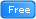* Java Database Connection: JDBC and MySQL
•Java JDBC with Oracle: Build a CRUD Application
•Java Object-Oriented Programming : Build a Quiz Application
•Understanding JDBC with PostgreSQL (A step by step guide)